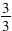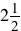##We are starting the first of several tasks on fractions. Some of this will be review, but it will help you be successful in later tasks. We are starting with writing fractions in lowest terms. This means that the numerator and the denominator do not have any common factors. Anytime you have a number over itself like this, it can be reduced to 1 (because this literally means three divided by three) . So we can cancel common factorsto write a fraction in lowest terms.

## Mixed and Improper Numbers

mixed number is a whole number and a fraction. You will see this frequently in a recipe. You may needcups of flour. This is an example of a mixed number.

An improper fractionis one where the numerator is greater than the denominator.

Let’s use our knowledge of the basics of fractions to perform operations with them.

When you add and subtract fractions with like denominators, you add the numerators and leave the denominators. What happens if we have unlike denominators? To add or subtract these fractions, we need the least common denominator . To find this we find the LCM of the denominators.

For example, if the denominators were 2 and 3, the LCM would be 6.

• We would multiply the two fractions by factors of 6 so that each was an equivalent fraction with a denominator of 6.